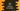# Different ways to find the factorial of a number in Java## Different ways to find the factorial of a number in Java:

In this post, we will learn different ways to find the factorial of a number in Java. The factorial of a number is the product of all numbers starting from 1 to that number. For example, the factorail of a number n is:

``1 * 2 * 3 *....* n``

! is used as the factorial symbol. For example, factorial of 4 is:

``4! = 1 * 2 * 3 * 4 = 24``

To find the factorial of a number, we have to use a loop. This loop will find the product of all numbers starting from 1 to n.

I will show you how to do it by using a for loop, while loop, do-while loop and by using a recursive method.

### Method 1: By using a for loop:

Let’s use a for loop to find the factorial:

``````import java.util.Scanner;

class Main {

public static int getFactorial(int n) {
int factorial = 1;
for (int i = 2; i <= n; i++) {
factorial *= i;
}

return factorial;
}

public static void main(String[] args) {
int num;

Scanner sc = new Scanner(System.in);
System.out.println("Enter the number: ");
num = sc.nextInt();

System.out.println("The factorial of " + num + " is: " + getFactorial(num));
}
}``````

Here,

• num is an integer variable to store the number.
• sc is a Scanner object to read the user input. It reads the input from the user and save it in the num variable.
• getFactorial method is used to calculate the factorial value of a number.

• It takes a number as the argument and returns the factorial.
• The for loop runs from 2 to the given number and multiply all values to another variable.
• It returns the factorial value.

If you run this program, it will give output as like below:

``````Enter the number:
12
The factorial of 12 is: 479001600

Enter the number:
6
The factorial of 6 is: 720

Enter the number:
5
The factorial of 5 is: 120``````

### Method 2: By using a while loop:

We can also use a while loop to write the above program.

``````import java.util.Scanner;

class Main {

public static int getFactorial(int n) {
int factorial = 1, i = 2;
while (i <= n) {
factorial *= i;
i++;
}

return factorial;
}

public static void main(String[] args) {
int num;

Scanner sc = new Scanner(System.in);
System.out.println("Enter the number: ");
num = sc.nextInt();

System.out.println("The factorial of " + num + " is: " + getFactorial(num));
}
}``````

I changed the for loop with a while loop. Both are almost similar. We are initializing the value of i before the loop starts. The loop runs until the value of i is less than or equal to n. At the end of each iteration, the value of i is incremented by 1.

If you run this program, it will give similar output.

### Method 3: By using a do-while loop:

We can also use a do-while loop. The difference between while and do-while loop is that while loop verifies the condition and then runs the code block. But, do-while loop runs the code and then verifies the condition.

If I replace the while loop with a do-while loop, it will look as like below:

``````import java.util.Scanner;

class Main {

public static int getFactorial(int n) {
int factorial = 1, i = 2;
do {
factorial *= i;
i++;
} while (i <= n);

return factorial;
}

public static void main(String[] args) {
int num;

Scanner sc = new Scanner(System.in);
System.out.println("Enter the number: ");
num = sc.nextInt();

System.out.println("The factorial of " + num + " is: " + getFactorial(num));
}
}``````

It will give similar output.

### Method 4: Recursive way:

Instead of using a loop, we can also use a recursive function. A recursive function calls itself repeatedly to find a result. In our case, it will call recursively to find the factorial.

Below is the complete program:

``````import java.util.Scanner;

class Main {

public static int getFactorial(int n, int result) {
if (n == 1) {
return result;
}

return getFactorial(n - 1, result * n);
}

public static void main(String[] args) {
int num;

Scanner sc = new Scanner(System.in);
System.out.println("Enter the number: ");
num = sc.nextInt();

System.out.println("The factorial of " + num + " is: " + getFactorial(num, 1));
}
}``````

For this example,

• getFactorial method takes two parameters, the first one is the number and the second one is another integer value to store the result.
• If the value of n is equal to 1, it returns the result value. Else, it calls the same method again and returns what it return.
• On each recursive call, it decrements the value of n by 1 and multiplies the value of result by n. At one point, the value of n will be 1 and it will return the current result value.